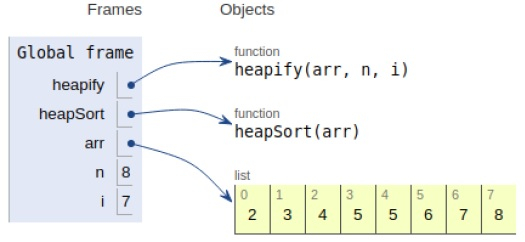# Python Program for Heap Sort

Problem statement − We are given an array, we need to sort it using the concept of heapsort.

Here we place the maximum element at the end. This is repeated until the array is sorted.

Now let’s observe the solution in the implementation below−

## Example

Live Demo

# heapify
def heapify(arr, n, i):
largest = i # largest value
l = 2 * i + 1 # left
r = 2 * i + 2 # right
# if left child exists
if l < n and arr[i] < arr[l]:
largest = l
# if right child exits
if r < n and arr[largest] < arr[r]:
largest = r
# root
if largest != i:
arr[i],arr[largest] = arr[largest],arr[i] # swap
# root.
heapify(arr, n, largest)
# sort
def heapSort(arr):
n = len(arr)
# maxheap
for i in range(n, -1, -1):
heapify(arr, n, i)
# element extraction
for i in range(n-1, 0, -1):
arr[i], arr = arr, arr[i] # swap
heapify(arr, i, 0)
# main
arr = [2,5,3,8,6,5,4,7]
heapSort(arr)
n = len(arr)
print ("Sorted array is")
for i in range(n):
print (arr[i],end=" ")

## Output

Sorted array is
2 3 4 5 5 6 7 8All the variables are declared in the local scope and their references are seen in the figure above.

## Conclusion

In this article, we have learned about how we can make a Python Program for Heap Sort